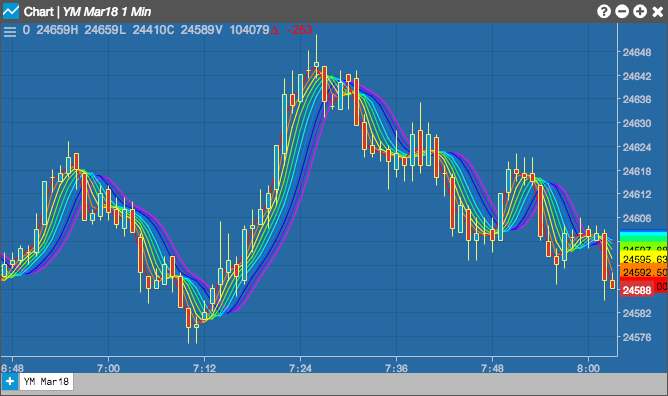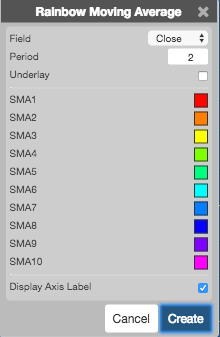Charts

# Rainbow Moving Average

The Rainbow Moving Average indicator shows multiple simple moving averages (SMAs) all at once for a specific time period. Each SMA is calculated based on the previous SMA and is color-coded in the chart.## Configuration Options• Field: Price or combination of prices to use as the base for average calculations. Possible values include:
• Open
• High
• Low
• Close
• HL/2 $$\left ( \frac{High + Low}{2} \right )$$
• HLC/3 $$\left ( \frac{High + Low + Close}{3} \right )$$
• HLCC/4 $$\left ( \frac{High + Low + Close + Close}{4} \right )$$
• OHLC/4 $$\left ( \frac{Open + High + Low + Close}{4} \right )$$
$Simple = MA = \frac{\sum_{i=1}^{n} Close_{i}}{n}$